In the previous blog, I generated a graph to show that a constant voltage active load driven by a high impedance source (in this case 240 volts RMS 50 Hz supply in series with a 3 Henry inductor) could establish one of two voltages to draw a particular power (in this case 18 watts). Here is the graph.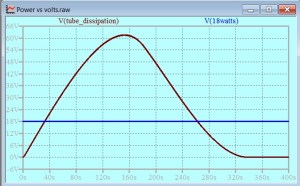See the previous post for details. I really wanted to show this on a graph with current vs. Voltage axes, where the constant power line would be an hyperbola and it would cross the load line in two places. Here I set out to do this.

Readers of this blog are likely to be familiar with the notion that the vector voltage across a resistor, and the vector voltage across a reactance in series, provide us with the wherewithal to construct a circular locus. In reflecting on this task, I have realized that in fact there are two different circles, and that we choose the one we want according to context. In small signal circuits, the reactance is most often a capacitor, but an inductive reactance shares the same critical property: the vector voltage is at right angles with the vector voltage across a resistor in series. Consider this circuit: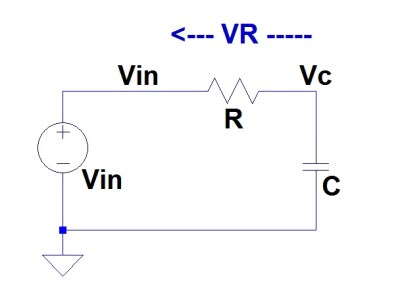The voltage across the resistor, VR and the voltage across the capacitor VC add to give the input voltage Vin. (Kirchhoff, or something, isn’t it?) Vectorially, the addition of voltages can be represented thus: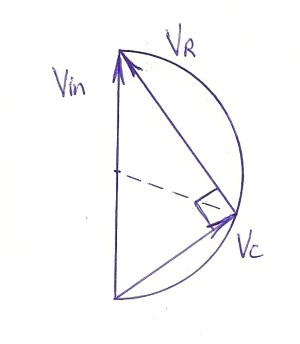As we learned in high school maths, “The angle in a semicircle is a right angle”. This vector (phasor) diagram is lovely to reflect on. It does not matter what the relative proportions of VR and VC are, the radius (shown dashed) of the circle to the point of connection between the vectors is constant. The relationship between the proportions of the vectors might vary with frequency as the reactance varies with frequency: here we have the genisis of an all-pass filter. On the other hand, if the radius is held fixed and at right angles to the input voltage by feedback, we have half a bridge that might form the basis of an audio oscillator.

I realized that if the supply was a constant AC voltage with an inductor in series, then a circular or elliptical load line would arise for a resistive load.Such a circle, (or an ellipse, which is just a circle with different scales in the orthogonal directions) does turn up on a load line for a source that has a reactive load. Here is a diagram from the Radiotron Designer’s Handbook – this is a loadline for instantaneous values: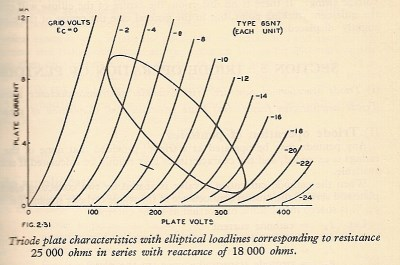This elliptical load line is for a resistance and a reactance in series. Exactly the same ellipse would be traversed by a capacitative reactance and an inductive reactance, but the instantaneous voltage on the anode of that triode would follow the ellipse in different directions in the two cases (anti-clockwise for a capacitor, and clockwise for an inductor).

The semicircle shown in the first diagram might have seemed to be defined (in part) by the relative angles of the vectors. However the same triangle could have been constructed with lines representing the magnitudes of scalars, such as the RMS values of voltages. Here is where we discover that there are two different circles generated by the very same vectors.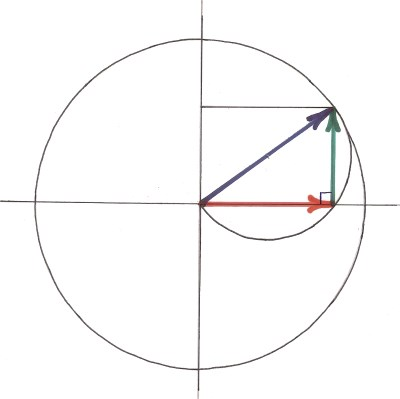Here, the supply voltage (blue vector) is shown as the sum of the resistor volt drop (red) and the inductor volt drop (green). The semicircle that we considered above is shown with (as above) the supply voltage being a diameter. If we show the vectors on Cartesian coordinates where the resistive drop and the inductive drop are shown on some axes, rather than just in free space and not relating to anything but each other, we see that the sum of them falls on a circle centered at the origin, and that the sum (the input voltage) is a radius of this circle.

This representation can be applied to RMS values, even though they are scalars. We can form the triangle with vectors with prior knowledge that the inductor volt drop and the resistor volt drop can be represented as vectors at right angles. Once we have the magnitudes of Input Volts, Inductor Volts, and Resistor volts, we can place lines representing them on “Inductor Volts vs Resistor Volts” axes, and produce a triangle that is similar (in the formal sense) to the vector one. If we normalize the inductive reactance to the value 1 then the inductor will pass one amp for every volt across it. The above circle diagram can then represent the inductor current (which is the same as the resistor current for a series circuit, or just “The current”) on the vertical axis, and the resistor volts on the horizontal axis. The “Load Line” for the high impedance supply is then a quarter of the big circle. We can do this without normalizing the inductive reactance, but the curve will then be an ellipse.

In the last post,(q.v.)  I drew the diagram Tube dissipation vs Volts – (reporoduced at the top of this post). The issue at hand was how the dissipation of a load would vary with the voltage across that load when the supply was a 240 volt RMS voltage provided via a 3H inductor. There were other complications: you would have to read that post. I wanted to see this picture on Current vs. Voltage axes, but the complications made this tricky. Here is a simplified case. the load is resistive. It is connected in series with a 3H inductor to a 240 v RMS supply. This is NOT a loadline for instantaneous values. Each point on these axes represents averaged (RMS is the average chosen) values for the whole cycle of the alternating waveform. Whereas in the instantaneous values loadline (above) the independent variable is the grid voltage, in the graph below, each point on the blue line represents a different value for the resistance.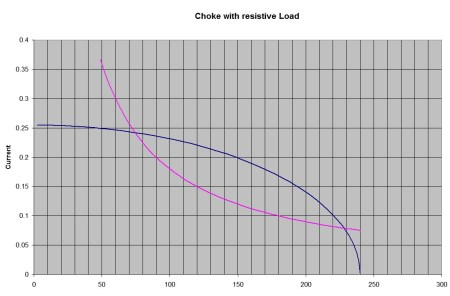The violet line represents a constant power of 18 watts. This is, of course an hyperbola on these axes. The blue line represents the power available to the resistor as the resistance is varied. the left-most intersection of these lines is the point for a low voltage electronic circuit. At about 75 volts in this case.